Data analysts often fit a probability distribution to data. When you have access to the data, a common technique is to use maximum likelihood estimation (MLE) to compute the parameters of a distribution that are "most likely" to have produced the observed data. However, how can you fit a distribution if you do not have access to the data?

This question was asked by a SAS programmer who wanted to fit a gamma distribution by using sample quantiles of the data. In particular, the p[rogrammer said, "we have the 50th and 90th percentile" of the data and "want to find the parameters for the gamma distribution [that fit] our data."

This is an interesting question. Recall that the method of moments uses sample moments (mean, variance, skewness,...) to estimate parameters in a distribution. When you use the method of moments, you express the moments of the distribution in terms of the parameters, set the distribution's moments equal to the sample moments, and solve for the parameter values for which the equation is true.

In a similar way, you can fit a distribution matching quantiles: Equate the sample and distributional quantiles and solve for the parameters of the distribution. This is sometimes called quantile-matching estimation (QME). Because the quantiles involve the cumulative distribution function (CDF), the equation does not usually have a closed-form solution and must be solved numerically.

### Fit a two-parameter distribution from two quantiles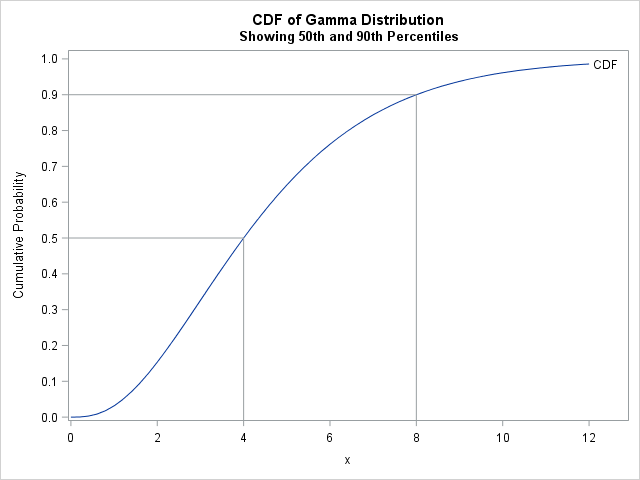To answer the programmer's question, suppose you do not have the original data, but you are told that the 50th percentile (median) of the data is x = 4 and the 90th percentile is x = 8. You suspect that the data are distributed according to a gamma distribution, which has a shape parameter (α) and a scale parameter (β). To use quantile-matching estimation, set F(4; α, β) = 0.5 and F(8; α, β) = 0.9, where F is the cumulative distribution of the Gamma(α, β) distribution. You can then solve for the values of (α, β) that satisfy the equations. You will get a CDF that matches the quantiles of the data, as shown to the right.

I have previously written about four ways to solve nonlinear equations in SAS. One way is to use PROC MODEL, as shown below:

```data initial; alpha=1; beta=1; /* initial guess for finding root */ p1=0.5; X1 = 4; /* eqn for 1st quantile: F(X1; alpha, beta) = p1 */ p2=0.9; X2 = 8; /* eqn for 2nd quantile: F(X2; alpha, beta) = p2 */ run;   proc model data=initial; eq.one = cdf("Gamma", X1, alpha, beta) - p1; /* find root of eq1 */ eq.two = cdf("Gamma", X2, alpha, beta) - p2; /* and eq2 */ solve alpha beta / solveprint out=solved outpredict; run;quit;   proc print data=solved noobs; var alpha beta; run;```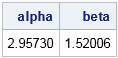The output indicates that the parameters (α, β) = (2.96, 1.52) are the values for which the Gamma(α, β) quantiles match the sample quantiles. You can see this by graphing the CDF function and adding reference lines at the 50th and 90th percentiles, as shown at the beginning of this section. The following SAS code creates the graph:

```/* Graph the CDF function to verify that the solution makes sense */ data Check; set solved; /* estimates of (alpha, beta) from solving eqns */ do x = 0 to 12 by 0.2; CDF = cdf("gamma", x, alpha, beta); output; end; run;   title "CDF of Gamma Distribution"; title2 "Showing 50th and 90th Percentiles"; proc sgplot data=Check; series x=x y=CDF / curvelabel; dropline y=0.5 X=4 / dropto=both; /* first percentile */ dropline y=0.9 X=8 / dropto=both; /* second percentile */ yaxis values=(0 to 1 by 0.1) label="Cumulative Probability"; xaxis values=(0 to 12 by 2); run;```

### Least squares estimates for matching quantiles

The previous section is relevant when you have as many sample quantiles as parameters. If you have more sample quantiles than parameters, then the system is overconstrained and you probably want to compute a least squares solution. If there are m sample quantiles, the least squares solution is the set of parameters that minimizes the sum of squares Σim (piF(xi; α, β))2.

For example, the following DATA step contains five sample quantiles. The observation (p,q) = (0.1, 1.48) indicates that the 10th percentile is x=1.48. The second observation indicates that the 25th percentile is x=2.50. The last observation indicates that the 90th percentile is x=7.99. You can use PROC NLIN to find a least squares solution to the quantile-matching problem, as follows:

```data SampleQntls; input p q; /* p is cumul probability; q is p_th sample quantile */ datalines; 0.1 1.48 0.25 2.50 0.5 4.25 0.75 6.00 0.9 7.99 ;   /* least squares fit of parameters */ proc nlin data=SampleQntls /* sometimes the NOHALVE option is useful */ outest=PE(where=(_TYPE_="FINAL")); parms alpha 2 beta 2; bounds 0 < alpha beta; model p = cdf("Gamma", q, alpha, beta); run;   proc print data=PE noobs; var alpha beta; run;```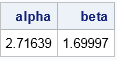The solution indicates the parameter values (α, β) = (2.72, 1.70) minimize the sum of squares between the observed and theoretical quantiles. The following graph shows the observed quantiles overlaid on the CDF of the fitted Gamma(α, β) distribution. Alternatively, you can graph the quantile-quantile plot of the observed and fitted quantiles.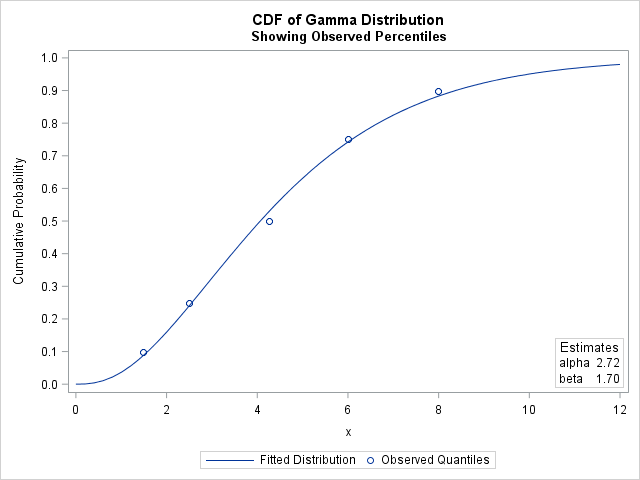### Weighted least squares estimates for matching quantiles

For small samples, quantiles in the tail of a distribution have a large standard error, which means that the observed quantile might not be close to the theoretical quantile. One way to handle that uncertainty is to compute a weighted regression analysis where each sample quantile is weighted by the inverse of its variance. According to Stuart and Ord (Kendall’s Advanced Theory of Statistics, 1994, section 10.10), the standard error of the p_th sample quantile in a sample of size n is σ2 = p(1-p) / (n fp)2), where ξp is the p_th quantile of the distribution and f is the probability density function.

In PROC NLIN, you can perform weighted analysis by using the automatic variable _WEIGHT_. The following statements define the variance of the p_th sample quantile and define weights equal to the inverse variance. Notice the NOHALVE option, which can be useful for iteratively reweighted least squares problems. The option eliminates the requirement that the weighted sum of squares must decrease at every iteration.

```/* weighted least squares fit where w[i] = 1/variance[i] */ proc nlin data=SampleQntls NOHALVE outest=WPE(where=(_TYPE_="FINAL")); parms alpha 2 beta 2; bounds 0 < alpha beta; N = 80; /* sample size */ xi = quantile("gamma", p, alpha, beta); /* quantile of distrib */ f = pdf("Gamma", xi, alpha, beta); /* density at quantile */ variance = p*(1-p) / (N * f**2); /* variance of sample quantiles */ _weight_ = 1 / variance; /* weight for each observation */ model p = cdf("Gamma", q, alpha, beta); run;```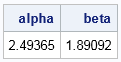The parameter estimates for the weighted analysis are slightly different than for the unweighted analysis. The following graph shows the CDF for the weighted estimates, which does not pass as close to the 75th and 90th percentiles as does the CDF for the unweighted estimates. This is because the PDF of the gamma distribution is relatively small for those quantiles, which causes the regression to underweight those sample quantiles.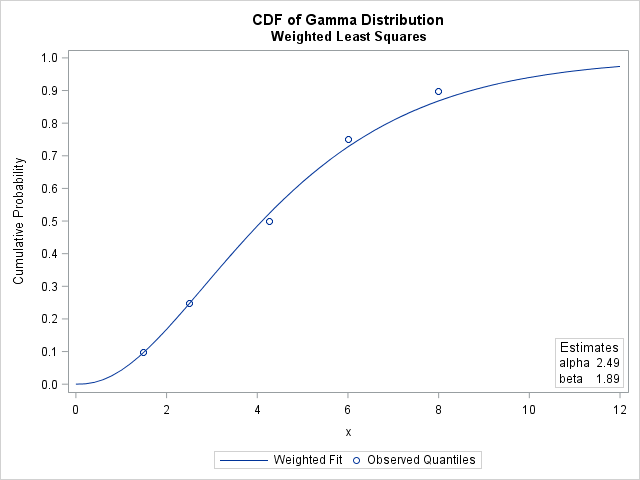In summary, this article shows how to use SAS to fit distribution parameters to observed quantiles by using quantile-matching estimation (QME). If the number of quantiles is the same as the number of parameters, you can numerically solve for the parameters for which the quantiles of the distribution equal the sample quantiles. If you have more quantiles than parameters, you can compute a least squares estimate of the parameters. Because quantile estimates in the tail of a distribution have larger uncertainty, you might want to underweight those quantiles. One way to do that is to run a weighted least squares regression where the weights are inversely proportional to the variance of the sample quantiles.

The post Fit a distribution from quantiles appeared first on The DO Loop.